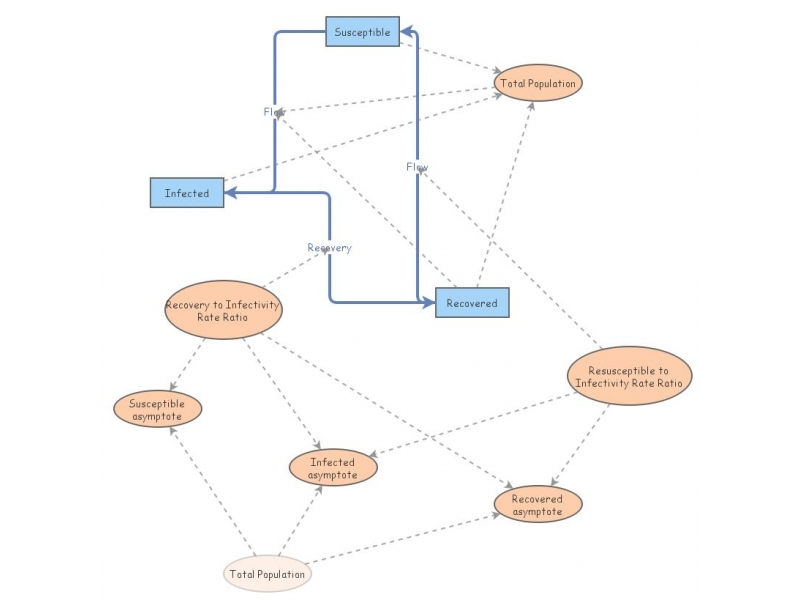A Simple, non-dimensionalized SIR (Susceptible, Infected, Recovered) model, with periodic infectivityThis is an example of an SIR (Susceptible, Infected, Recovered) model that has been re-parameterized down to the bare minimum, to illustrated the dynamics possible with the fewest number of parameters.

We're rescaled this SIR model, so that time is given in infection rate-appropriate time units, "rates" are now ratios of rates (with infectivity rate in the denominator), and populations are considered proportions (unfortunately InsightMaker doesn't function properly if I give them all values from 0 to 1, which sum to 1 -- so, at the moment, I give them values that sum to 100, and consider the results percentages).

The new display includes the asymptotics: the three sub-populations will tend to fixed values as time goes to infinity; the infected population goes to zero if the recovery rate is greater than the infectivity rate -- i.e., the disease dies out.

Note the use of a "ghost" stock (for Total Population), which I think is a pretty cool idea. It cuts down on the number of arcs in the model graph.

A comparable model in Mathematica is available at
http://www.nku.edu/~longa/classes/2018spring/mat375/mathematica/SIRModel-rescaled.nb